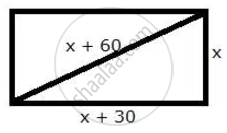Share

The Diagonal of a Rectangle is 60 M More than Its Shorter Side and the Larger Side is 30 M More than the Shorter Side. Find the Sides of the Rectangle. - ICSE Class 10 - Mathematics

Question

The diagonal of a rectangle is 60 m more than its shorter side and the larger side is 30 m more than the shorter side. Find the sides of the rectangle.

SolutionLet the shorter side be x m.
Length of the other side = (x + 30) m
Length of hypotenuse = (x + 60) m
Using Pythagoras theorem,
(x + 60)2 = x2 + (x + 30)2
x2 + 3600 + 120x = x2 + x2 + 900 + 60x
x2 – 60x – 2700 = 0
x2 – 90x + 30x – 2700 = 0
x(x – 90) + 30(x – 90) = 0
(x – 90) (x + 30) = 0
x = 90, -30
But, x cannot be negative. So, x = 90.
Thus, the sides of the rectangle are 90 m and (90 + 30) m = 120 m.

Is there an error in this question or solution?

APPEARS IN

Selina Solution for Selina ICSE Concise Mathematics for Class 10 (2018-2019) (2017 to Current)
Chapter 6: Solving (simple) Problems (Based on Quadratic Equations)
Ex.6B | Q: 5

Video TutorialsVIEW ALL 

Solution The Diagonal of a Rectangle is 60 M More than Its Shorter Side and the Larger Side is 30 M More than the Shorter Side. Find the Sides of the Rectangle. Concept: Quadratic Equations.
S